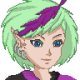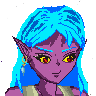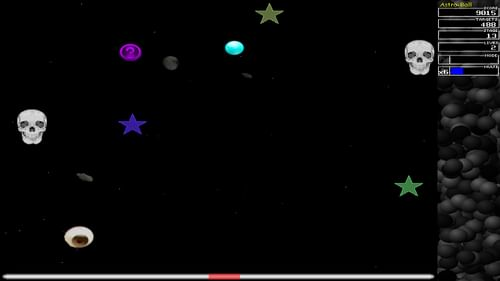-=+=- -=+=- -=+=- -=+=- -=+=- -=+=- -=+=- -=+=- -=+=- -=+=- -=+=- -=+=- -=+=- -=+=- -=+=- -=+=- -=+=- -=+=- -=+=- -=+=- -=+=- -=+=- -=+=- -=+=- -=+=- -=+=- -=+=- -=+=- -=+=- -=+=- (c) WidthPadding Industries 1987 0|93|0 -=+=- -=+=- -=+=- -=+=- -=+=- -=+=- -=+=- -=+=- -=+=- -=+=- -=+=- -=+=- -=+=- -=+=- -=+=- -=+=- -=+=- -=+=- -=+=- -=+=- -=+=- -=+=- -=+=- -=+=- -=+=- -=+=- -=+=- -=+=- -=+=- -=+=-### Tricky

View Profile, Send Private Message
Recent Posts, Showcases, Articles, Code Snippets, Links, Wed.Workshop, Blog

### Latest Showcases

Tricky# Kitty - Console based Code Viewer

21st April 2020Kitty is a console based code viewer, with syntax highlight. The name is a pun on the Unix tool ''cat''.

The screenshot was demonstrated in PowerShell, but that's merely because I prefer PowerShell above cmd, as it should work in cmd as well*snip*

Tricky# Kthura - Map Editor

24th October 2019Let's show you my Kthura Map Editor

Kthura is a map system for 2D Map development, but here's the rub... It's not a tile sysem, but a object oriented map system. I originally designed it for an adventure game with a girl named Kthura as main*snip*

Tricky# Astrobounce

18th October 2019Astrobounce